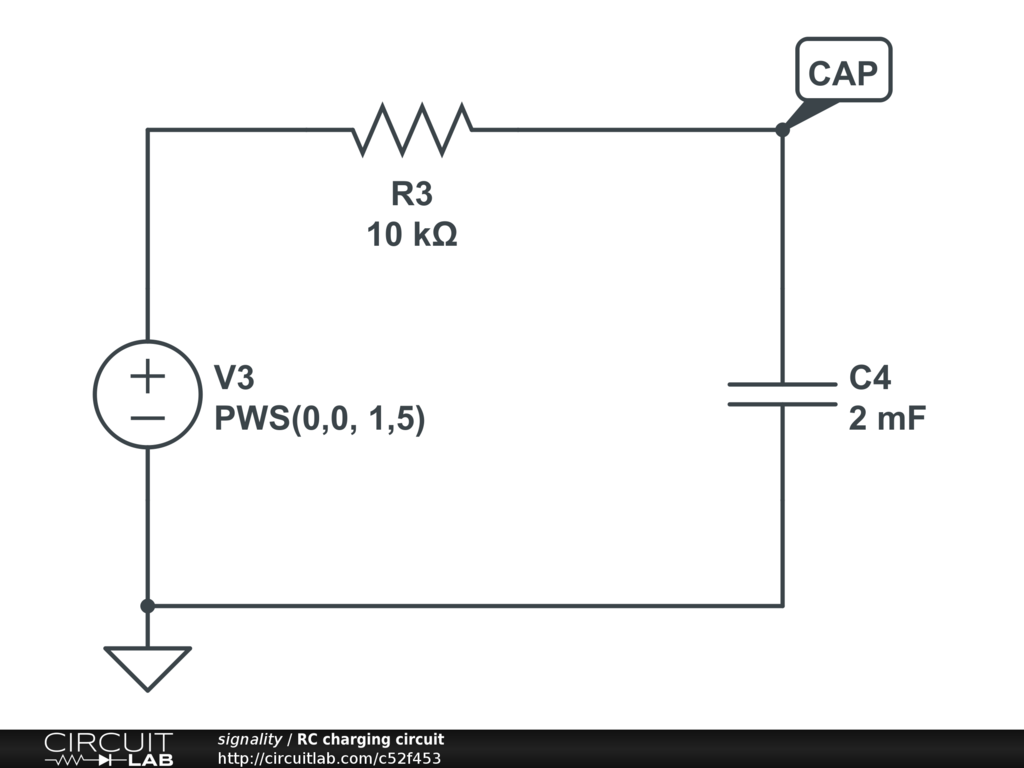## is circuit lab giving me the wrong plots?

 Hello so i'm new to circuits and circuit lab, i'm trying to simulate the following cricuit https://www.circuitlab.com/editor/gnv7p5/ but when i simulate it, the graph V vs t gives me a wrong answer, like the capacitor is discharging when it should be charging from the battery. Any idea why it happen? what am i doing wrong? how could i fix it? Thanks in advance. by kinoakuro October 23, 2015 I think you have misunderstood a few things about CL and possibly about how and where to probe circuits. i) You have used a DC source which in most simulation tools would be assumed to be generating 5V for all time including t < 0. So when you start the simulation at t =0, the capacitor is already charged up and therefore the voltage across the resistor would already be at zero and would stay there. However, CL has the poorly documented `Skip initial conditions` option. It is not clear exactly what this does but it seems that in your sim, it makes the 5V source = 0 for t < 0 and at t = 0 it sets the voltage source to 5V. Under these conditions, the caps start with zero charge, i.e. with zero volts across them. At the instant that V1 steps up to 5V the voltage across the caps stays at 0V so the voltage across R1 steps up to 5V. The voltage across R1 then decays exponentially to zero as the voltage across the caps exponentially increases to 5V. ii) To avoid this confusion in the voltage source initial conditions you could use the PWS() voltage source option to create a source that explicitly starts at 0V and then steps up to 5V some time t > 0. About the PWS() source: https://www.circuitlab.com/docs/expressions/ iii) If you want to see the voltage across the caps rising to 5V from zero then you need the ground at the supply end of the caps not at the supply end of R1. Or measure the the voltage difference across the caps. This:demonstrates what I think you are trying to achieve. :) by signality October 24, 2015 thank you very much, now it is clear for me! i apreciate it. by kinoakuro October 24, 2015